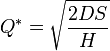# MM/U4 Topic 3 Dynamic-Certain EOQ Model

As the name suggests, Economic order quantity (EOQ) model is the method that provides the company with an order quantity. This order quantity figure is where the record holding costs and ordering costs are minimized. By using this model, the companies can minimize the costs associated with the ordering and inventory holding. In 1913, Ford W. Harris developed this formula whereas R. H. Wilson is given credit for the application and in-depth analysis on this model.

The economic order quantity (EOQ) is a model that is used to calculate the optimal quantity that can be purchased or produced to minimize the cost of both the carrying inventory and the processing of purchase orders or production set-ups.

#### Formula

Following is the formula for the economic order quantity (EOQ) model:Where Q = optimal order quantity

D = units of annual demand

S = cost incurred to place a single order or setup

H = carrying cost per unit

This formula is derived from the following cost function:

Total cost = purchase cost + ordering cost + holding cost

Limitations of the economic order quantity model:

It is necessary for the application of EOQ order that the demands remain constant throughout the year. It is also necessary that the inventory be delivered in full when the inventory levels reach zero.

Assumption of the EOQ model

Following are the underlying assumptions for the EOQ model. Without these assumptions, the EOQ model cannot work to its optimal potential.

• The cost of the ordering remains constant.
• The demand rate for the year is known and evenly spread throughout the year.
• The lead time is not fluctuating (lead time is the latency time it takes a process to initiate and complete).
• No cash or settlement discounts are available, and the purchase price is constant for every item.
• The optimal plan is calculated for only one product.
• There is no delay in the replenishment of the stock, and the order is delivered in the quantity that was demanded, i.e. in whole batch.
• These underlying assumptions are the key to the economic order quantity model, and these assumptions help the companies to understand the shortcomings they are incurring in the application of this model.

## One thought on “MM/U4 Topic 3 Dynamic-Certain EOQ Model”

error: Content is protected !!